## Example Questions

← Previous 1 3 4 5

### Example Question #1 : How To Find Data Representation In Physics

Mary is performing an experiment involving the electromagnetic spectrum. She observes several different types of waves and records their wavelength, frequency, and speed.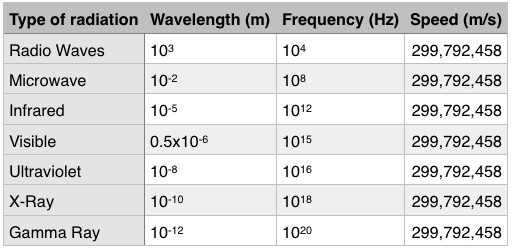Which type of radiation has the greatest wavelength?

Ultraviolet light

Visible light

Gamma rays

Explanation:

The chart provides the waves in descending order of wavelength. Radio waves has a wavelength of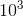, much greater than the other answer choices. Remember that a positive exponent will always be greater than a negative exponent. Furthermore, the smaller a negative exponent is, the bigger its value.### Example Question #2 : How To Find Data Representation In Physics

A student was interested in determining the relationship between the current, voltage, and resistance in a direct circuit, such as those exemplified by batteries connected to light bulbs. The student built the circuit presented in Figure 1 using a 2 ohm resistor.

Figure 1: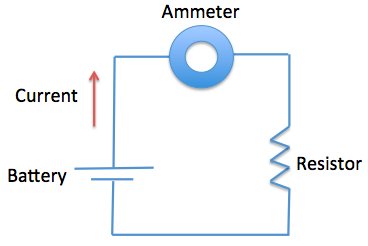The current that flows through the circuit can be calculated using the equation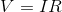, whereis the voltage of the battery,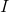is the current flowing through the circuit, andis the resistance of the resistor.

The student used a 2 ohm resistor and batteries of various voltages to obtain the results in Table 1. The currents shown in the table are NOT calculated using the formula, but instead directly measured from the circuit using an ammeter. It is important to note that the measured current will only exactly equal the calculated current if the system contains no internal resistance.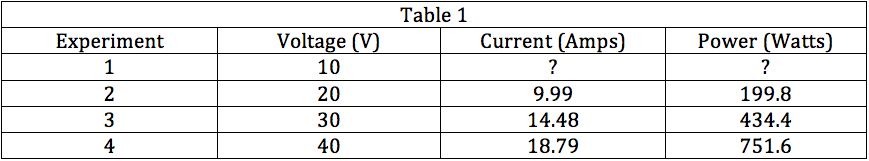Based on the formula provided in the passage and the data from the experiments, if voltage increases, current __________.

remains the same

increases

decreases

drops to zero

increases

Explanation:

Given the formula, we can see that both voltage and current are in the numerator of their respective side of the equal sign; thus, these values are directly related and if voltage increases, current will also increase. This is supported by the data from the experiments.

### Example Question #3 : How To Find Data Representation In Physics

A student was interested in determining the relationship between the current, voltage, and resistance in a direct circuit, such as those exemplified by batteries connected to light bulbs. The student built the circuit presented in Figure 1 using a 2 ohm resistor.

Figure 1:The current that flows through the circuit can be calculated using the equation, whereis the voltage of the battery,is the current flowing through the circuit, andis the resistance of the resistor.

The student used a 2 ohm resistor and batteries of various voltages to obtain the results in Table 1. The currents shown in the table are NOT calculated using the formula, but instead directly measured from the circuit using an ammeter. It is important to note that the measured current will only exactly equal the calculated current if the system contains no internal resistance.Which of the following shows a possible graphical relationship between current and voltage?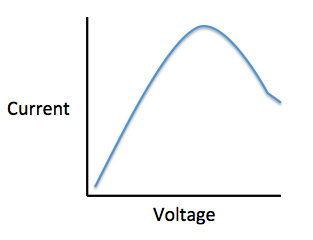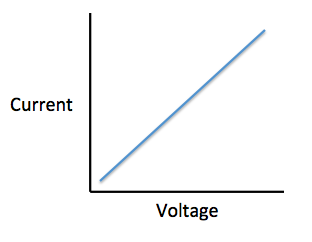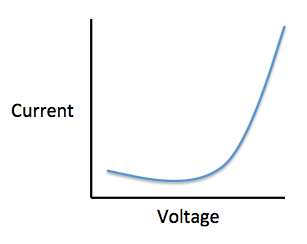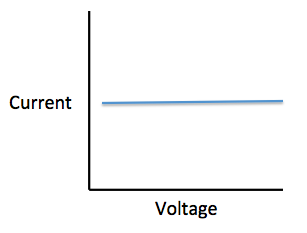Explanation:

Using the formula, we see that voltage and current are directly related, meaning that as one increases, the other increases proportionally. The only graph that gives us this linear relationship is.

### Example Question #4 : How To Find Data Representation In Physics

A student was interested in determining the relationship between the current, voltage, and resistance in a direct circuit, such as those exemplified by batteries connected to light bulbs. The student built the circuit presented in Figure 1 using a 2 ohm resistor.

Figure 1:The current that flows through the circuit can be calculated using the equation, whereis the voltage of the battery,is the current flowing through the circuit, andis the resistance of the resistor.

The student used a 2 ohm resistor and batteries of various voltages to obtain the results in Table 1. The currents shown in the table are NOT calculated using the formula, but instead directly measured from the circuit using an ammeter. It is important to note that the measured current will only exactly equal the calculated current if the system contains no internal resistance.Which of the following shows a possible graphical relationship between current and power?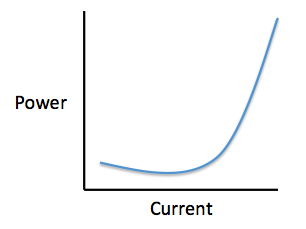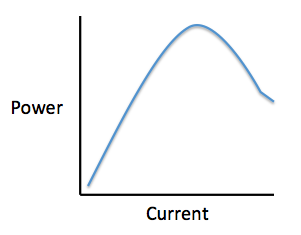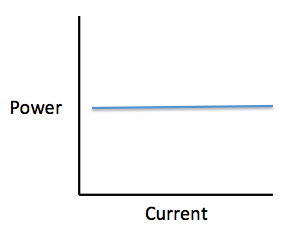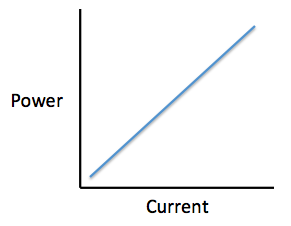Explanation:

Using the formula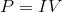, we see that current and power are directly related, meaning that as one increases, the other increases proportionally. The only graph that gives us this linear relationship is.

### Example Question #5 : How To Find Data Representation In Physics

A student was interested in determining the relationship between the current, voltage, and resistance in a direct circuit, such as those exemplified by batteries connected to light bulbs. The student built the circuit presented in Figure 1 using a 2 ohm resistor.

Figure 1:The current that flows through the circuit can be calculated using the equation, whereis the voltage of the battery,is the current flowing through the circuit, andis the resistance of the resistor.

The student used a 2 ohm resistor and batteries of various voltages to obtain the results in Table 1. The currents shown in the table are NOT calculated using the formula, but instead directly measured from the circuit using an ammeter. It is important to note that the measured current will only exactly equal the calculated current if the system contains no internal resistance.In Experiment 1, how much current is most likely flowing through the circuit?

5 amps

1 amp

12 amps

10 amps

5 amps

Explanation:

The passage provides us with a formula to calculate the amount of current running through the circuit,. We are told the voltage in Experiment 1 is 10 V and the resistor is 2 ohms, so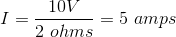.

### Example Question #6 : How To Find Data Representation In Physics

The photoelectric effect is a phenomenon that has led to many important scientific discoveries. Light of a particular wavelength is shined onto a piece of metal, showering the metal with photons. Wavelength is inversely related to a photon's energy. That is, with a smaller wavelength, the photon has greater energy. The wavelength of the light is decreased until a detector next to the metal senses that electrons are being ejected from the metal. This sensor also tells us how many electrons are ejected per second, which we call electrical current. At this point, any additional decrease in wavelength does not affect the number of electrons ejected. This point is called the metal's work function. However, if we then begin to increase the intensity of the light being shone (meaning the amount of light as opposed to the light's wavelength), the number of electrons picked up by the sensor increases.

Which of the following graphs comparing photon energy (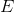) and amount of electrons ejected (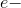) would best represent the information provided in the passage?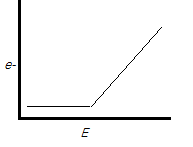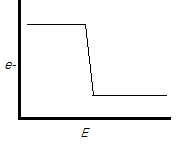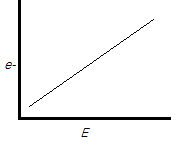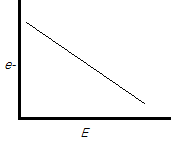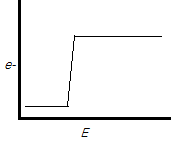Explanation:

The correct answer is. As the passage says, the energy increases until suddenly the metal begins to emit a stream of electrons. At this point, as the passage states, the number of electrons does not change, explaining the plateau we see in this graph. The other graphs are not consistent with this information.

### Example Question #7 : How To Find Data Representation In Physics

Sound waves travel through a medium by mechanically disturbing the particles of that medium. As particles in the medium are displaced by the sound wave, they in turn act upon neighboring particles. In this fashion, the wave travels through the medium through a parallel series of disturbed particles. Like in other forms of motion, the rate at which the sound wave travels can be measured by dividing the distance over which the wave travels by the time required for it to do so.

Study 1
A group of students hypothesizes that the velocity of sound is dependent upon the density of the medium through which it passes. They propose that with more matter in a given space, each particle needs to travel a shorter distance to disturb the adjacent particles. Using two microphones and a high speed recording device, the students measured the delay from the first microphone to the second. They chose a variety of media, shown in Table 1, and measured the velocity of sound through each using their two-microphone setup. The results are found in Table 1.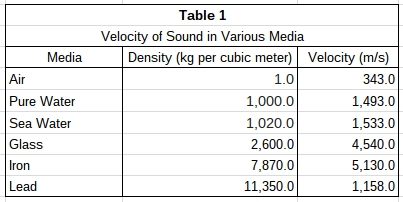Study 2
The students wanted to test their hypothesis by using the same medium at different densities. To do this, they heated pure water to various temperatures and repeated the procedure described in Study 1. Their results can be found in Table 2.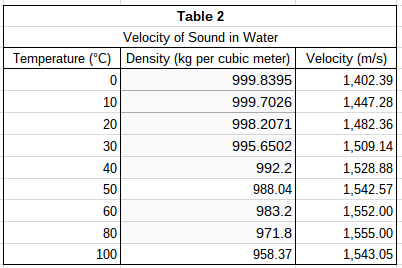According to Study 2, over what temperature interval does velocity begin to decrease as water temperature rises?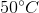to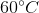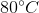to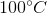to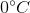to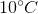toExplanation:

Table 2 lists velocity of sound in its third column. As you move down the column, each value corresponds to increasing temperatures. The velocity values increase over each interval until they drop from 1,555 m/s to 1543.05 m/s. This decrease in velocity occurs between 80°C and 100°C according to the corresponding values in the first column of the table.

### Example Question #8 : How To Find Data Representation In Physics

Sound waves travel through a medium by mechanically disturbing the particles of that medium. As particles in the medium are displaced by the sound wave, they in turn act upon neighboring particles. In this fashion, the wave travels through the medium through a parallel series of disturbed particles. Like in other forms of motion, the rate at which the sound wave travels can be measured by dividing the distance over which the wave travels by the time required for it to do so.

Study 1
A group of students hypothesizes that the velocity of sound is dependent upon the density of the medium through which it passes. They propose that with more matter in a given space, each particle needs to travel a shorter distance to disturb the adjacent particles. Using two microphones and a high speed recording device, the students measured the delay from the first microphone to the second. They chose a variety of media, shown in Table 1, and measured the velocity of sound through each using their two-microphone setup. The results are found in Table 1.Study 2
The students wanted to test their hypothesis by using the same medium at different densities. To do this, they heated pure water to various temperatures and repeated the procedure described in Study 1. Their results can be found in Table 2.According to the data in Study 1, as density increases, what happens to the velocity of sound?

It decreases.

It does not change.

It increases.

It either increases or decreases.

It either increases or decreases.

Explanation:

The velocity of sound in different media is listed in the third column of Table 1. Density of the media increases as you move down the column. Velocity increases along with density except between iron and lead. This means that no direct relationship between density and velocity can be drawn; the velocity of sound can either increase or decrease as density increases.

### Example Question #1321 : Act Science

Sound waves travel through a medium by mechanically disturbing the particles of that medium. As particles in the medium are displaced by the sound wave, they in turn act upon neighboring particles. In this fashion, the wave travels through the medium through a parallel series of disturbed particles. Like in other forms of motion, the rate at which the sound wave travels can be measured by dividing the distance over which the wave travels by the time required for it to do so.

Study 1
A group of students hypothesizes that the velocity of sound is dependent upon the density of the medium through which it passes. They propose that with more matter in a given space, each particle needs to travel a shorter distance to disturb the adjacent particles. Using two microphones and a high speed recording device, the students measured the delay from the first microphone to the second. They chose a variety of media, shown in Table 1, and measured the velocity of sound through each using their two-microphone setup. The results are found in Table 1.Study 2
The students wanted to test their hypothesis by using the same medium at different densities. To do this, they heated pure water to various temperatures and repeated the procedure described in Study 1. Their results can be found in Table 2.In Study 1, which medium tested had the greatest density?

Air

Glass

Iron

Sea Water

Explanation:

The densities for the media in Study 1 can be found in Table 1. In the second column of Table 1, the densities are listed in ascending order. Lead has the highest number in that column and therefore has the greatest density.

### Example Question #1322 : Act Science

Sound waves travel through a medium by mechanically disturbing the particles of that medium. As particles in the medium are displaced by the sound wave, they in turn act upon neighboring particles. In this fashion, the wave travels through the medium through a parallel series of disturbed particles. Like in other forms of motion, the rate at which the sound wave travels can be measured by dividing the distance over which the wave travels by the time required for it to do so.

Study 1
A group of students hypothesizes that the velocity of sound is dependent upon the density of the medium through which it passes. They propose that with more matter in a given space, each particle needs to travel a shorter distance to disturb the adjacent particles. Using two microphones and a high speed recording device, the students measured the delay from the first microphone to the second. They chose a variety of media, shown in Table 1, and measured the velocity of sound through each using their two-microphone setup. The results are found in Table 1.Study 2
The students wanted to test their hypothesis by using the same medium at different densities. To do this, they heated pure water to various temperatures and repeated the procedure described in Study 1. Their results can be found in Table 2.According to Study 2, water at which of the following temperatures yields the greatest velocity of sound?